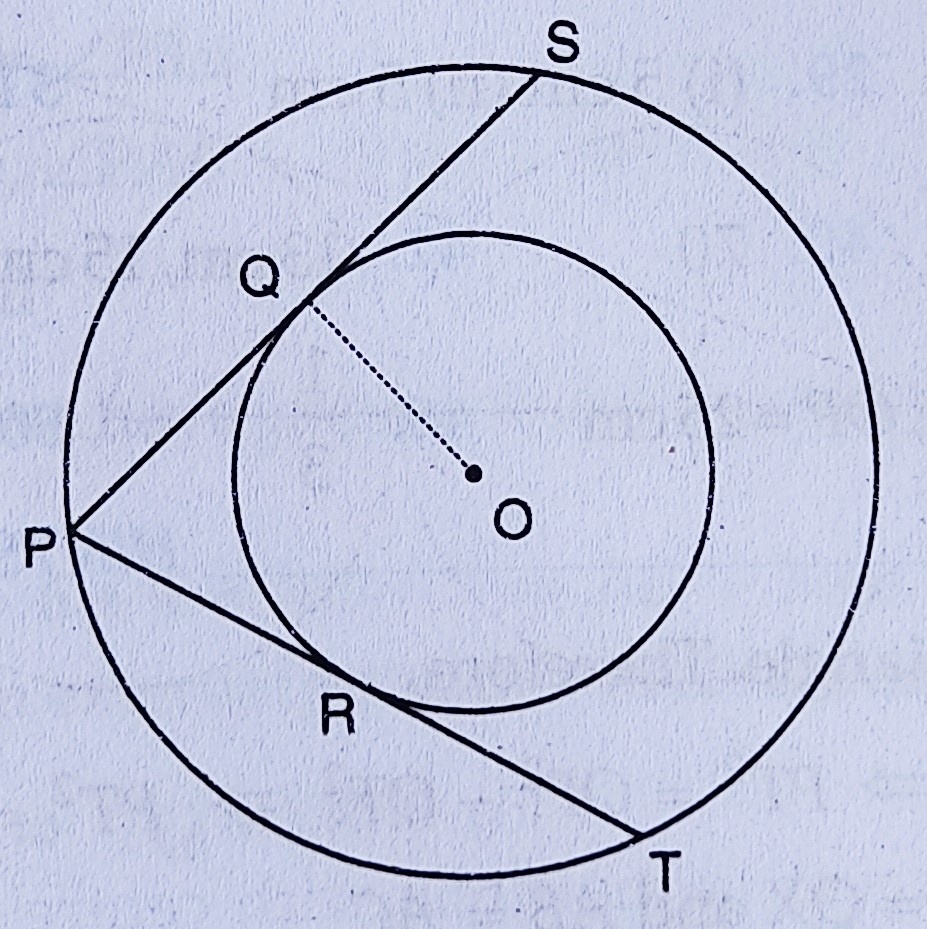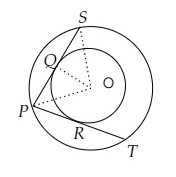# In the figure, there are two concentric circles with centre O. $P R T$ and $P Q S$ are tangents to the inner circle from a point $P$ lying on the outer circle. If $P R=5 \mathrm{~cm}$, find the length of $P S$."

Given:

In the figure, there are two concentric circles with centre O. $P R T$ and $P Q S$ are tangents to the inner circle from a point $P$ lying on the outer circle.

$P R=5 \mathrm{~cm}$.

To do:
We have to find the length of $P S$.

Solution:Join $OS$ and $OP$.

In $\triangle POS$,

$PO = OS$

This implies,

$\triangle POS$ is an isosceles triangle.

We know that,

In an isosceles triangle, if a line drawn perpendicular to the base of the triangle from the common vertex of the equal sides, then that line will bisect the base.

$PQ = PR = 5\ cm$   ($PRT$ and $PQS$ are tangents to the inner circle from a point $P$ lying on the outer circle)

$PQ = QS$

$PQ = 5\ cm$

$QS = 5\ cm$

From the figure,

$PS = PQ + QS$

$PS = 5 + 5$

$PS = 10\ cm$

The length of $P S$ is $10\ cm$.